# Operational Amplifier-Basic Concepts and Applications

The operational amplifier (also known as Op-Amp) is a multi-terminal direct coupled high gain amplifier which consisting of one or more differential amplifiers and a level transistor and an output.

The Op-Amp is a versatile device which can be used to amplify both DC and AC signals and these are mainly designed for performing mathematical operations like addition, subtraction, multiplication etc.

## Block Diagram of Operational Amplifier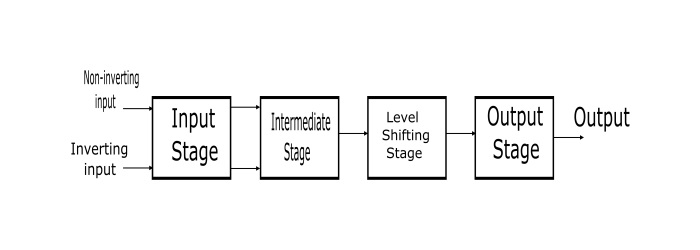• Input Stage − The input stage is the dual input, balanced output differential amplifier. This stage provides most of the voltage gain and introduces the input resistance of operational amplifier.

• Intermediate Stage − This stage is dual input, unbalanced output differential amplifier, which is driven by the output of first stage.

• Level Shifting Stage − Since direct coupling is used, therefore the DC voltage at the output of intermediate stage is above the ground potential. Hence, the level shifting transistor circuit is used after intermediate stage to shift the DC level at intermediate stage output downward to zero volts with respect to ground.

• Output Stage − The output stage is a push-pull complementary amplifier. The output stage increases the output voltage. The output stage also provides low output resistance.

## Schematic Symbol of Op-Amp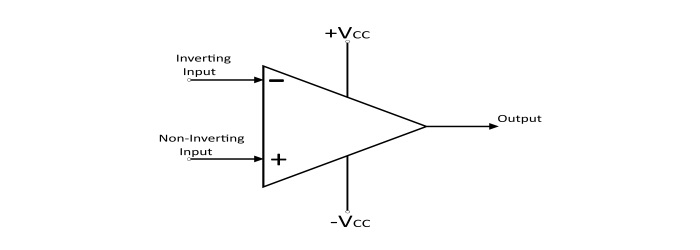Operational amplifiers have three main terminals: two high input impedance inputs and one low impedance output. The non-inverting input terminal is denoted by positive sign and the inverting input terminal is denoted by a negative sign. Other than input-output terminals there are two Op-Amp power supply terminal denoted by (+VCC) and (-VCC).

## Working Principle of Operational Amplifier

### Open Loop Operation

In the open loop operation there is no feedback provided in the amplifier circuit. Therefore, two signals one at inverting terminal and the other at the non-inverting terminal applied then, the Op-Amp amplifies the difference of the two applied signals. This difference of the two input signals is called as differential input voltage.

The output of Op-Amp is given by

$$\mathrm{V_{out}=A_{open\:loop}(V_{1}-V_{2})}$$

Where,

$$\mathrm{V_{out}=output\:voltage}$$

$$\mathrm{A_{open\:loop}=Open\:Loop\:gain\:of\:Op-Amp}$$

$$\mathrm{V_{1}=Voltage\:at\:the\:non\:inverting\:terminal}$$

$$\mathrm{V_{2}=Voltage\:at\:the\:inverting\:terminal}$$

$$\mathrm{(V_{1}-V_{2})=Differential\:input\:voltage}$$

The output of the Op-Amp is non-zero only when the differential input voltage is non-zero i.e. V1 and V2 are not equal. The open loop gain (A) of Op-Amp is very high. Thus an open loop Op-Amp can amplify a small differential input signal to a high value. The Op-Amp can amplify the input signal to a very high value but cannot exceed the supply voltage of operational amplifier.

### Close Loop Operation

When the Op-Amp provided with a feedback signal is known as closed loop operational amplifier. The feedback path feeds the output signal to the input.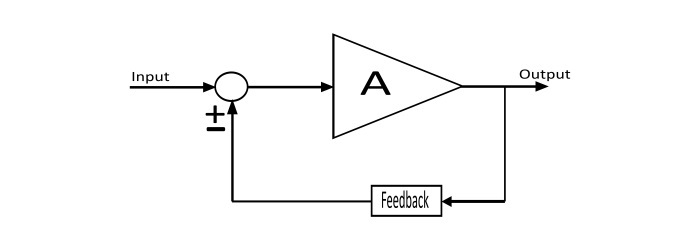The output equation for the closed loop operation is given by

$$\mathrm{V_{out}=A_{close\:loop}(V_{1}-V_{2})}$$

When the feedback is connected to non-inverting terminal then feedback is called as the positive feedback. The positive feedback is used in oscillator applications.

When the feedback is connected to inverting terminal then it is called as negative feedback. The negative feedback is used the amplification applications.

## Characteristics of Ideal Operational Amplifier

An ideal Op-Amp would exhibit the following electrical characteristics −

• Infinite voltage gain (A).

• Infinite input resistance (Ri) so that any source of signal can drive it.

• Zero output resistance (Ro) so that output can drive infinite number of other devices.

• Infinite bandwidth so that signal of any frequency (from 0 to ∞ Hz) can be amplified.

• Infinite Common Mode Rejection Ration (CMRR = ∞) so the output common mode noise voltage is zero.

• Infinite slew rate so that output voltage changes simultaneously with the input voltage changes

## Equivalent Circuit of Operational Amplifier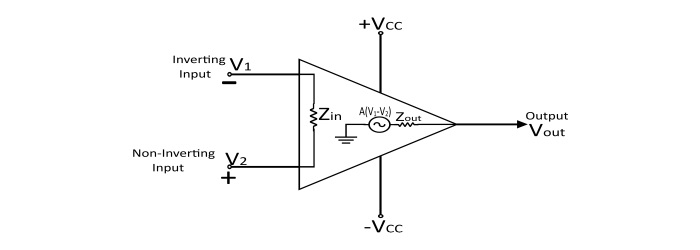The integrated Op-Amp has the following advantages −

• High Reliability
• Small size and cheap
• Power consumption is less

## Applications of Operational Amplifier

Op-Amp are most commonly used in following applications −

• Voltage Follower• Inverting Amplifier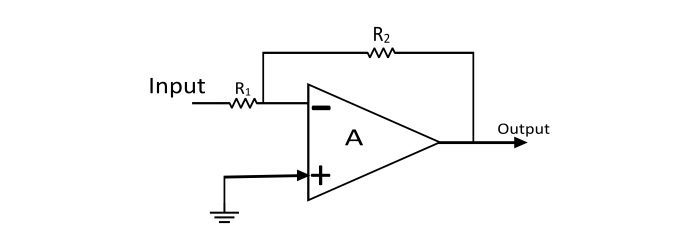• Non-Inverting Amplifier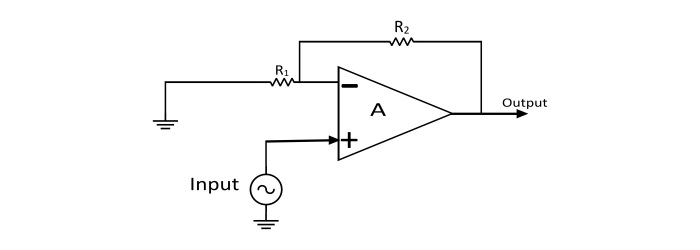• Other useful applications of Op-Amp are − Summing Amplifier, Current to Voltage Converter, Voltage to Current Converter, Differential Amplifier, Integrator and Differentiator, Instrumentation Amplifier, Log Amplifier, Antilog or Exponential Amplifier, Precision Diode, HWR and FWR etc.